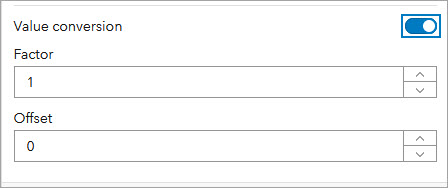# Convert values

For the gauge and indicator elements, authors can indicate that numbers should be converted from one unit to another. This is helpful when the data is in a certain set of units, but you want to display it in another set of units without changing it. There are many specific use cases for this:

• Length conversions (for example, miles to kilometers)
• Weight conversions (for example, pounds to kilograms)
• Temperature conversions (for example, degrees Celsius to Fahrenheit)
• Power conversions (for example, watts to kilowatts)
• Number conversions (for example, decimal to percent)

In general, value conversion can be represented by the equation y = mx + b. Using this formula, a number can be converted to any other number along a straight line.

• x = number to be converted
• m = conversion factor (slope) to be applied to x
• b = offset to be applied (y-axis intercept)
• y = number that is displayed in the dashboard

The Value conversion setting is on the Data tab for the indicator and gauge. After you enable Value Conversion, two additional settings appear: Factor and Offset.The Factor setting represents the m in the equation y = mx + b and the Offset setting represents the b.

The following table provides examples of numbers to enter in the Factor and Offset settings to perform value conversions.

 Type of conversion Factor value Offset value Number—Decimal to percent 100 Leave as 0 Temperature—Degrees Celsius to degrees Fahrenheit 1.8 32 Weight—Pounds to kilograms 0.45359237 Leave as 0

You can't enter fractions in the Factor setting (for example, 5/9). You can only enter positive and negative decimals and whole numbers.